## 从赌钱游戏看PageRank算法 原荐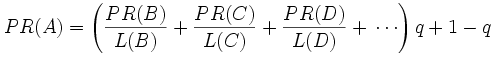double x1=1.0,x2=1.0,x3=1.0;

double x2_ income =x1/2.0;
double x3_ income =x1/2.0+x2;
double x1_ income =x3;

// Gamble单机程序

``````public class Gamble
{
public static double x1=1.0,x2=1.0,x3=1.0;

public static void playgame(){
double x2_income=x1/2.0;
double x3_income=x1/2.0+x2;
double x1_income=x3;
x1=x1_income;
x2=x2_income;
x3=x3_income;
System.out.println("x1:"+x1+", x2:"+x2+", x3:"+x3);
}

public static void main(String[] args){
for(int i=0;i<500;i++){
System.out.print("第"+i+"轮 ");
playgame();
}
}
}``````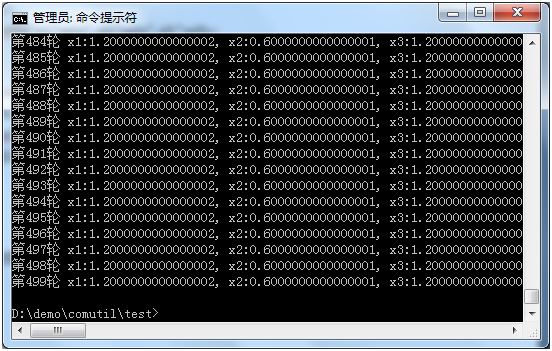x1:1.2000000000000002, x2:0.6000000000000001, x3:1.2000000000000002
…...

double x2_income=x1/2.0+x3/2.0;
double x3_income=x1/2.0+x2;
double x1_income=x3/2.0;

x1:0.6666666666666667, x2:1.0, x3:1.3333333333333333

double x2_income=x1/2.0+x3/2.0;
double x3_income=x1/2.0+x2;
double x1_income=0;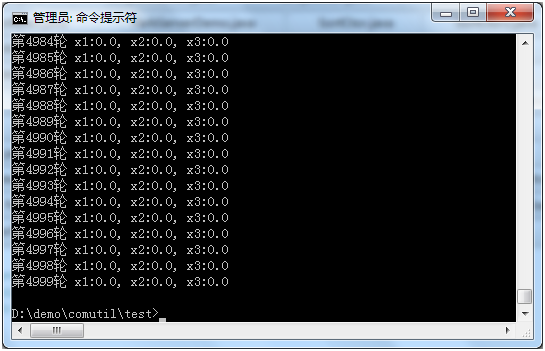A包含B和C的链接
B包含C的链接
C包含A的链接

PR(B)=PR(A)/2;
PR(C)=PR(A)/2+PR(B);
PR(A)=PR(C);

``````PR(x1)+ PR(x2)+ …+PR(xn)
=( (PR(x2)/ L(x2)+ … )*q+1-q) + … + ( (PR(x1)/ L(x1)+ … )*q+1-q)
=(PR(x1)* L(x1)/L(x1) + PR(x2)* L(x2)/L(x2) + … + PR(xn)* L(xn)/L(xn))q + n(1-q)
=( PR(x1) + PR(x2) + … + PR(xn))*q + n - n*q
=n*q + n – n*q
= n``````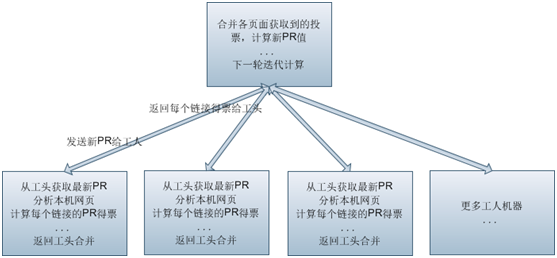PageRankWorker：是一个PageRank工人实现，为了方便演示，它通过一个字符串数组代表包括的链接（实际上应该从本地网页文件里获取）
links = new String[]{"B","C"};

pagepr = pagepr+(Double)prwh.getObj(page);

1、 启动ParkServerDemo（它的IP端口已经在配置文件指定）
java -cp fourinone.jar; ParkServerDemo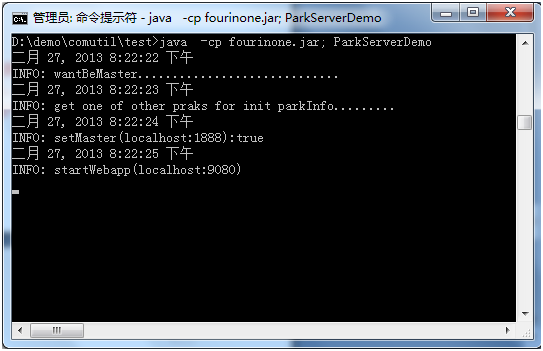2、运行A、B、C三个PageRankWorker，传入不同的IP和端口号
java  -cp fourinone.jar; PageRankWorker localhost 2008 A
java  -cp fourinone.jar; PageRankWorker localhost 2009 B
java  -cp fourinone.jar; PageRankWorker localhost 2010 C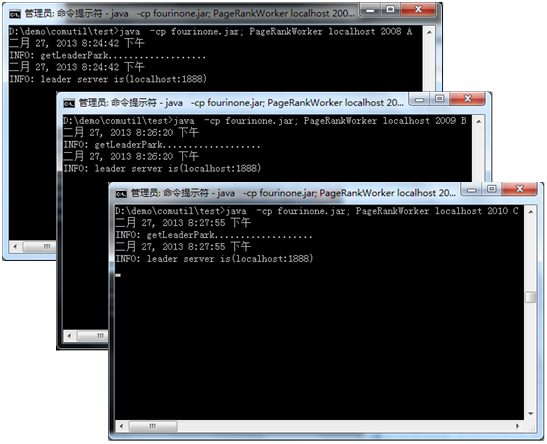3、运行PageRankCtor
java -cp fourinone.jar; PageRankCtor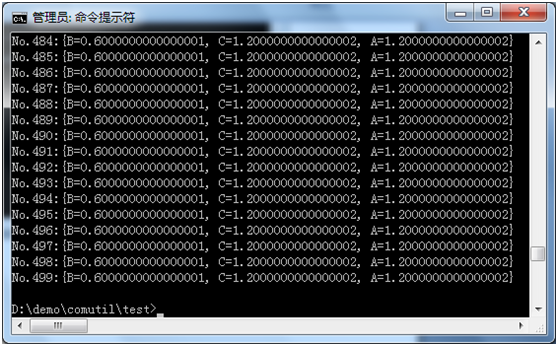// ParkServerDemo

``````import com.fourinone.BeanContext;
public class ParkServerDemo
{
public static void main(String[] args)
{
BeanContext.startPark();
}
}``````

// PageRankWorker

``````import com.fourinone.MigrantWorker;
import com.fourinone.WareHouse;
import com.fourinone.Workman;

public class PageRankWorker extends MigrantWorker
{
public String page = null;
public String[] links = null;

public PageRankWorker(String page, String[] links){
this.page = page;
}

public WareHouse doTask(WareHouse inhouse)
{
Double pr = (Double)inhouse.getObj(page);
System.out.println(pr);

WareHouse outhouse = new WareHouse();

return outhouse;
}

public static void main(String[] args)
{
String[] links = null;
if(args.equals("A"))
links = new String[]{"B","C"};//A页面包括的链接
else if(args.equals("B"))
links = new String[]{"C"};
else if(args.equals("C"))
links = new String[]{"A"};

PageRankWorker mw = new PageRankWorker(args,links);
mw.waitWorking(args,Integer.parseInt(args),"pagerankworker");
}
}``````

// PageRankCtor
``````import com.fourinone.Contractor;
import com.fourinone.WareHouse;
import com.fourinone.WorkerLocal;
import java.util.Iterator;

public class PageRankCtor extends Contractor
{
public WareHouse giveTask(WareHouse inhouse)
{
WorkerLocal[] wks = getWaitingWorkers("pagerankworker");
System.out.println("wks.length:"+wks.length);

for(int i=0;i<500;i++){//500轮
WareHouse[] hmarr = doTaskBatch(wks, inhouse);
WareHouse prwh = new WareHouse();
for(WareHouse result:hmarr){
for(Iterator iter=result.keySet().iterator();iter.hasNext();){
String page = (String)iter.next();
Double pagepr = (Double)result.getObj(page);
if(prwh.containsKey(page))
pagepr = pagepr+(Double)prwh.getObj(page);
prwh.setObj(page,pagepr);
}
}
inhouse = prwh;//迭代
System.out.println("No."+i+":"+inhouse);
}
return inhouse;
}

public static void main(String[] args)
{
PageRankCtor a = new PageRankCtor();
WareHouse inhouse = new WareHouse();
inhouse.setObj("A",1.00d);//A的pr初始值
inhouse.setObj("B",1.00d);//B的pr初始值
inhouse.setObj("C",1.00d);//C的pr初始值
a.exit();
}
}``````### fourinone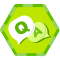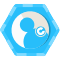### 评论(19)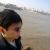pagerank 的数学理论基础 好像就是来自 马可夫链...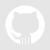#### 引用来自“呆阿呆”的评论

( (PR(x2)/ L(x2)+ … )*q+1-q) + … + ( (PR(x1)/ L(x1)+ … )*q+1-q)
=(PR(x1)* L(x1)/L(x1) + PR(x2)* L(x2)/L(x2) + … + PR(xn)* L(xn)/L(xn))q + n(1-q)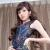#### 引用来自“zqhxuyuan”的评论

PR(B)=PR(A)/2;
PR(B)=PR(A)/2+PR(C);
PR(A)=PR(C);

#### 引用来自“奥特曼FFF”的评论

( (PR(x2)/ L(x2)+ … )*q+1-q) + … + ( (PR(x1)/ L(x1)+ … )*q+1-q)
=(PR(x1)* L(x1)/L(x1) + PR(x2)* L(x2)/L(x2) + … + PR(xn)* L(xn)/L(xn))q + n(1-q)

PageRank原理、举例、实现及使用networkX库简单调用

nana-li
2018/07/27
0
0

monkey_d_meng
2011/06/19
0
0

2018/05/10
0
0
pagerank 算法 快速入门

yuejiewc
2018/05/23
0
0
PageRank算法原理与实现

1 PageRank 1.1 简介 PageRank，又称网页排名、谷歌左侧排名，是一种由搜索引擎根据网页之间相互的超链接计算的技术，而作为网页排名的要素之一，以Google公司创办人拉里·佩奇（Larry Page）...

2018/07/20
0
0

linux shell使用代理

0
0
Krpano 插入视频-video

hotspot-video <hotspot name="videospot" url="%SWFPATH%/plugins/videoplayer.swf" alturl="%SWFPATH%/plugins/videoplayer.js" videourl="%SWFPATH%/image/video.flv|%......

3分钟前
0
0
el-select使用方法及遇到数据回显的坑

<el-select v-model="temp.lang" class="filter-item" placeholder="Please select"> <el-option v-for="item in langs" :key="item.value" :label="item.label" :value="item.value"/> </el......

BraveLN
5分钟前
0
0

MrPei
10分钟前
0
0
windows nginx

miaojiangmin
15分钟前
0
0